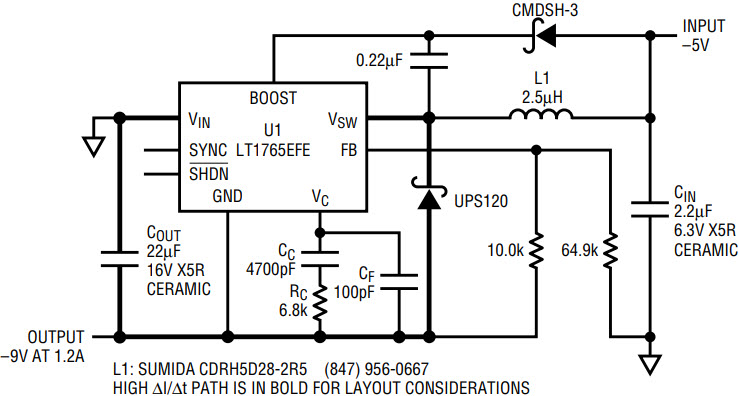# Positive Buck Regulator Makes Negative Boost DC/DC Converter

Power supply designers can choose from a plethora of available positive buck regulators that can also be used as negative boost DC/DC converters. Some buck regulators have a negative feedback reference voltage expressly for this purpose, but they are far outnumbered by the variety of ICs that have positive reference feedback voltages. A designer can take advantage of this greater variety of devices by using a positive buck switch-mode regulator to create an excellent negative boost converter—all that is needed are a few small modifications to the typical buck converter configuration.

Figure 1a shows a –5V input to –9V output at 1.2A negative boost converter using the LT1765EFE positive buck converter switch-mode regulator. The LT1765EFE operates with a 3V to 25V input, uses a 1.2V feedback voltage, and has an internal 3A power switch. The fast 1.25MHz switching frequency of the LT1765EFE helps reduce the size of the inductor and input and output capacitors. Figure 1b shows a typical positive buck converter application for the LT1765EFE, a 12V in to 3.3V out at 2.2A DC/DC converter.Figure 1a. –5V input to –9V output at 1.2A DC/DC converter.Figure 1b. 12V input to 3.3V output at 2.2A DC/DC converter.

In Figure 1a the VIN pin is connected to system ground and the GND pin of the IC is connected to the negative voltage output. This makes the negative boost converter configuration provide a positive voltage at the VFB pin with respect to the GND pin of the IC. In this topology the maximum input voltage rating of the IC has to be greater than the magnitude of output voltage for the negative boost converter. The IC must also have a minimum input voltage rating that is less than the magnitude of the input voltage in order for the circuit to turn-on upon power-up, since the output voltage can have an initial state of 0V.Figure 2. Efficiency of the negative boost converter in Figure 1a is as high as 85% and typically greater than 80%.

Notice that the maximum output current for the negative boost converter in Figure 1a is less than the maximum output current of the positive buck converter Figure 1b, even though they use the same 3A internal power switch.

Inductor Selection

The inductor is chosen based on maximum output current, peak switch current, and desired ripple current. First calculate the duty cycle (DC), and then either calculate the ripple current (IP–P) based on the chosen inductor (L), or the inductor value based on the desired ripple current. It is generally good practice to choose the inductor value so that the peak-to-peak ripple current is about 40% of the input current. These calculations are approximate and ignore the effect of switch, inductor, and Schottky diode power losses.

where η is the overall efficiency

where f is the switching frequency

Maximum inductor current (IL(MAX)) is equal to peak switch current in this configuration. The IC has a maximum switch current (ISW(MAX)) of 3A, so the maximum inductor current must remain below 3A. To keep switch current below the maximum, more inductance might be needed to keep the ripple current low enough.

Maximum output current (IOUT(MAX)) is an approximation derived from the maximum allowable input current given the ripple current.

Input and Output Capacitors

Like a typical boost converter, the input capacitor in the negative boost topology has low ripple current and the output capacitor has high discontinuous ripple current. The size of the output capacitor is typically bigger than the input capacitor in order to handle the greater RMS ripple current.

The output capacitor ESR has a direct effect on the output voltage ripple of the DC/DC converter. Choosing higher frequency switch-mode regulators reduces the need for excessive RMS ripple current rating. Regardless, a low-ESR output capacitor, such as a ceramic, can minimize the output voltage ripple of the negative boost converter.

Layout

Figures 1a and 1b show the high ΔI/Δt switching paths of the negative boost and positive buck DC/DC converters. This loop must be kept as small as possible, by minimizing trace lengths, in order to minimize trace inductance. The discontinuous currents in this path create very high ΔI/Δt values. Any trace inductance in this loop results in voltage spikes that can render a circuit noisy or uncontrollable. For this reason, circuit layout can be just as important as component selection. Note that the layout of the negative boost is similar to the positive buck regulator, with the locations of the input and output swapped.